deutsch     english    français     Print

## 5.2 INTELLIGENT ROBOTS### INTRODUCTION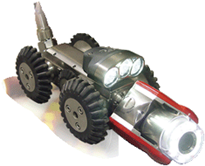Robots that can find their way in a changing environment have many potential applications, for example as flying objects, in underwater exploration, and in the examination of sewer systems. Here you will learn step by step how you can build a moving robot that is able to orient itself in a changing environment

 PROGRAMMING CONCEPTS: Externally controlled, autonomous, self-learning robot, teach mode, execution mode, event loop

### THE ROBOT KNOWS THE WAY

 In the simplest case, a robot should be able to find a path in a very special canal that consists of elements of the same length arranged orthogonally. Information about the constant length of the canal elements and whether they consist of left or right curves is hardcoded ("wired") in the program.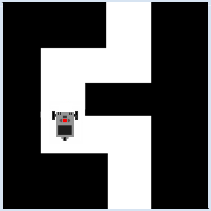```from simrobot import * #from nxtrobot import * #from ev3robot import * RobotContext.useObstacle("sprites/bg.gif", 250, 250) RobotContext.setStartPosition(310, 470) moveTime = 3200 turnTime = 545 robot = LegoRobot() gear = Gear() robot.addPart(gear) gear.forward(moveTime) gear.left(turnTime) gear.forward(moveTime) gear.right(turnTime) gear.forward(moveTime) gear.right(turnTime) gear.forward(moveTime) gear.left(turnTime) gear.forward(moveTime) robot.exit() ``` Highlight program code (Ctrl+C to copy, Ctrl+V to paste)

### MEMO

 You have to figure out moveTime and turnTime through a series of experiments and then adjust accordingly. Naturally, they correlate to the speed of the robot. In reality, you would probably rather specify the route to be traversed and the rotation angles instead of the times.

### ROBOT CONTROLLED BY A HUMAN

The robot knows the constant lengths of the canal elements, but its turning movements are controlled by a human. However, the robot is not capable of learning and it cannot remember the path, so it remains "stupid".
To control the robot in both the simulation and external control mode, you use the left and right cursor keys of the keyboard, and in autonomous mode you use the corresponding LEFT and RIGHT buttons. With the methods isLeftHit() and isRightHit() you can ask whether the keys or the buttons were pressed and again released. Use the escape key or ESCAPE button to exit the program.
 ```from simrobot import * #from nxtrobot import * #from ev3robot import * RobotContext.useObstacle("sprites/bg.gif", 250, 250) RobotContext.setStartPosition(310, 470) moveTime = 3200 turnTime = 545 robot = LegoRobot() gear = Gear() robot.addPart(gear) gear.forward(moveTime) while not robot.isEscapeHit(): if robot.isLeftHit(): gear.left(turnTime) gear.forward(moveTime) if robot.isRightHit(): gear.right(turnTime) gear.forward(moveTime) robot.exit() ``` Highlight program code (Ctrl+C to copy, Ctrl+V to paste)

### MEMO

 In this case, it makes less sense to use the autonomous mode since you actually want to remote control the robot. You can also use the infrared remote control for this with the EV3 instead of using the keyboard (see Additional Material at the end of this chapter).

### THE ROBOT LEARNS IN TEACH MODE

Computer-aided systems, whose behavior is not hardcoded and who can therefore later adapt their behavior to an environment, are called adaptive systems. These are therefore capable of learning, in a way. Industrial robots are “trained” by specialists in a "teach mode", for instance which arm movements are to be carried out. In most cases, the operator uses an input system similar to a remote control. The robot is successively moved to the desired positions and the respective state is stored.

In "execution mode" the robot runs through the stored states independently (and with a higher speed).As before, your canal robot knows the constant length of the canal elements, but its turning movements are controlled by a human. However, the robot is now able to learn, so it can memorize the path and independently run through it any number of times. It is often useful to imagine that in every moment, a robot is in a particular state. The states are typically labeled with meaningful words and stored as a string. You assume the following states: the robot is stopped, moving forward, turning left, or right, and you call them: STOPPED, FORWARD, LEFT, RIGHT. [more... It would be better to use an enumerated type (enum) for the states. In the version of Python on the EV3 but they are missing] Instead of constantly querying the keys or buttons, here you use a more elegant event programming model with registered callback functions, which are, independently of the currently running program, always called automatically when an event occurs. The main program, in an endless loop, is engaged in performing the corresponding actions in each state. The state change takes place in the callback onButtonHit().
 ```from simrobot import * #from nxtrobot import * #from ev3robot import * RobotContext.useObstacle("sprites/bg.gif", 250, 250) RobotContext.setStartPosition(310, 470) RobotContext.showStatusBar(30) def onButtonHit(buttonID): global state if buttonID == BrickButton.ID_LEFT: state = "LEFT" elif buttonID == BrickButton.ID_RIGHT: state = "RIGHT" elif buttonID == BrickButton.ID_ENTER: state = "RUN" moveTime = 3200 turnTime = 545 memory = [] robot = LegoRobot(buttonHit = onButtonHit) gear = Gear() robot.addPart(gear) state = "FORWARD" while not robot.isEscapeHit(): if state == "FORWARD": robot.drawString("Moving forward", 0, 3) gear.forward(moveTime) state = "STOPPED" robot.drawString("Teach me!", 0, 3) elif state == "LEFT": memory.append(0) robot.drawString("Saved: LEFT-TURN", 0, 3) gear.left(turnTime) state = "FORWARD" elif state == "RIGHT": memory.append(1) robot.drawString("Saved: RIGHT-TURN", 0, 3) gear.right(turnTime) state = "FORWARD" elif state == "RUN": robot.drawString("Executing memory", 0, 1) robot.drawString(str(memory), 0, 2) robot.reset() robot.drawString("Moving forward", 0, 3) gear.forward(moveTime) for k in memory: if k == 0: robot.drawString("Turning left", 0, 3) gear.left(turnTime) else: robot.drawString("Turning right", 0, 3) gear.right(turnTime) robot.drawString("Moving forward", 0, 3) gear.forward(moveTime) gear.stop() robot.drawString("All done", 0, 3) state = "STOPPED" robot.exit() ``` Highlight program code (Ctrl+C to copy, Ctrl+V to paste)

### MEMO

 Processing the states occurs in a while loop in the main part of the program, instead of in callback functions. In computer science, such a loop is commonly called an event loop. Only the corresponding state is selected in the callback (state switch). With this programming technique, you can obtain a clear chronological synchronization between the longer lasting actions and the call of the event-driven callback, which can occur at any given time. The "memory" consists of a list where you store the numbers 0 or 1, depending on whether the path branches off to the left or the right.

### THE SELF-LEARNING ROBOT

In certain applications it is not possible for the robot to be taught by an operator. The robot could, for example, be located somewhere outside of the immediate communication area (e.g. on Mars).

In order to find the path, the robot now has to capture the environment using built-in sensors and “act” accordingly. People know the environment mainly from seeing with their eyes. For robots, image capture with a camera is easy, but the analysis of these images can get extremely complicated [more... The evaluation of images belongs to the science of pattern recognition].

In order to orient itself in the canal, your robot uses only a touch sensor that will trigger an event when pressed. The canal should always consist of canal elements of equal lengths. When the robot receives a touch event after passing through a canal member, it knows that it is at a turning point in the path. It then goes back a bit and tries to progress with a left turn.
If within a short amount of time it bumps into a wall again, it knows that it took the wrong path. It then goes back again and this time moves to the right. The robot remembers whether it had to turn to the right or to the left in order to go the right way, and it can later run through the canal any number of times on its own without bumping into a wall.

Let the robot run through the canal in teach mode. Then, you press the enter key or the ENTER button in order to transfer it from teach mode into execution mode.
 ```from simrobot import * #from nxtrobot import * #from ev3robot import * import time RobotContext.useObstacle("sprites/bg.gif", 250, 250) RobotContext.setStartPosition(310, 470) RobotContext.showStatusBar(30) def onPressed(port): global startTime global backTime robot.drawString("Press event!", 0, 1) dt = time.clock() - startTime # time since last hit in s gear.backward(backTime) if dt > 2: memory.append(0) gear.left(turnTime) # turning left else: memory.pop() memory.append(1) gear.right(2 * turnTime) # turning right robot.drawString("Mem: " + str(memory), 0, 1) gear.forward() startTime = time.clock() def run(): for k in memory: robot.drawString("Moving forward", 0, 1) gear.forward(moveTime) if k == 0: robot.drawString("Turning left", 0, 1) gear.left(turnTime) elif k == 1: robot.drawString("Turning right", 0, 1) gear.right(turnTime) gear.forward(moveTime) robot.drawString("All done", 0, 1) moveTime = 3200 turnTime = 545 backTime = 700 memory = [] robot = LegoRobot() gear = Gear() robot.addPart(gear) ts = TouchSensor(SensorPort.S3, pressed = onPressed) robot.addPart(ts) startTime = time.clock() gear.forward() robot.drawString("Moving forward", 0, 1) while not robot.isEscapeHit(): if robot.isEnterHit(): robot.reset() run() robot.exit() ``` Highlight program code (Ctrl+C to copy, Ctrl+V to paste)

### MEMO

 The touch sensor is connected to the Port S3 (in the simulation mode, this corresponds to an assembly position in the middle front). The sensor signals the touch events via the callback onPressed(), which is registered using the named parameter press. The touch sensor is a robot component that is added to the robot as usual with addPart(). To find out whether the robot has moved into either a canal element or a dead end, determine the time since the last touch event using the built-in Python clock. If it is more than two seconds, the robot moved forward into a canal element, otherwise it was a dead end. Since the robot always turns to the left first and writes a 0 in its brain, in the case of a dead end it has to substitute the incorrect 0 with a 1.

### THE ENVIRONMENT GETS MORE COMPLEX

You have probably noticed that the robot is perfectly able to figure out by itself how far forward it has to go until the next turn occurs, since it can measure the time that it takes until it bumps into the end of the canal element. This way, the robot is able to move appropriately in a canal with differing lengths of canal elements as well. However, now it must not only keep the left-right information in its head, but also the moving time. You best pack both related pieces of information together in a list node = [moveTime, k], where moveTime is the moving time (in ms), k = 0 is a left turn, and k = 1 is a right turn.

You will get moveTime after passing through the canal element, but you still have to correct it by the time by which the robot has driven too far. Then you store it in a global variable, since you will have to use it again in case the robot moved into a dead end.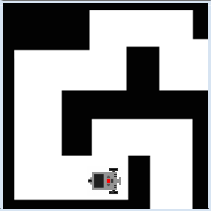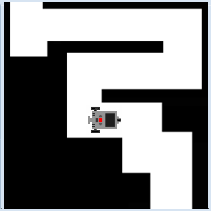```# Robo2e.py from simrobot import * #from nxtrobot import * #from ev3robot import * import time RobotContext.useObstacle("sprites/bg2.gif", 250, 250) RobotContext.setStartPosition(410, 460) RobotContext.showStatusBar(30) def pressCallback(port): global startTime global backTime global turnTime global moveTime dt = time.clock() - startTime # time since last hit in s gear.backward(backTime) if dt > 2: moveTime = int(dt * 1000) - backTime # save long-track time node = [moveTime, 0] memory.append(node) # save long-track time gear.left(turnTime) # turning left else: memory.pop() # discard node node = [moveTime, 1] memory.append(node) gear.right(2 * turnTime) # turning right robot.drawString("Memory: " + str(memory), 0, 1) gear.forward() startTime = time.clock() def run(): for node in memory: moveTime = node k = node robot.drawString("Moving forward",0, 1) gear.forward(moveTime) if k == 0: robot.drawString("Turning left",0, 1) gear.left(turnTime) elif k == 1: robot.drawString("Turning right",0, 1) gear.right(turnTime) gear.forward() # must stop manually robot.drawString("All done, press DOWN to stop", 0, 1) isExecuting = False turnTime = 545 backTime = 700 robot = LegoRobot() gear = Gear() robot.addPart(gear) ts = TouchSensor(SensorPort.S3, pressed = pressCallback) robot.addPart(ts) startTime = time.clock() moveTime = 0 memory = [] gear.forward() while not robot.isEscapeHit(): if robot.isDownHit(): gear.stop() elif robot.isEnterHit(): robot.reset() run() robot.exit() ``` Highlight program code (Ctrl+C to copy, Ctrl+V to paste)

### MEMO

 At first sight, the data structure of memory as a list of node lists may seem complicated. However, data belonging together (in this case, the running time of the next track and the subsequent left-right information) should always be stored in a shared data structure. Enjoy that, with the same program, the robot can also find its way in a completely different canal (e.g. bg3.gif).

### EXERCISES

1.
 The robot should independently pass through a canal shown on the right using a touch sensor, but without learning. For the simulation mode, use the following RobotContext options: ```RobotContext.useObstacle("sprites/bg2gif", 250, 250) RobotContext.setStartPosition(400, 470) ```2.

Write a program for a lawn mower robot using a touch sensor that mows the grass strip by strip. It bumps into the boundary of the lawn above and below.

 For the simulation mode, use the following RobotContext options: ```RobotContext.useBackground("sprites/fieldbg.gif") RobotContext.useObstacle("sprites/field1.gif", 250, 250) RobotContext.setStartPosition(350, 300) ```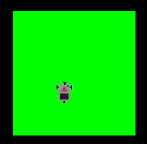3.

Unfortunately the boundary of the lawn now has a hole where the robot is able to escape. Create a program with a learning robot that knows how long the strips are after cutting the first strip of grass and thus no longer has to use the touch sensor.

 For the simulation mode, use the following RobotContext options: ```RobotContext.useBackground("sprites/fieldbg.gif") RobotContext.useObstacle("sprites/field2.gif", 250, 250) RobotContext.setStartPosition(350, 300) ```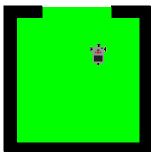### TEACH MODE WITH THE INFRARED REMOTE CONTROL

(only EV3 autonomous mode)

You've already learned in the last chapter, how to use the EV3 infrared remote control. Here you uses it to guide the robot in teach mode through the channel. Both in teach as in execute mode, the program runs autonomously on the EV3.

This approach is very close to reality as it is common in industrial robots, they "learn" with a remote control and then execute the "learned" program.

In the teach mode, you use the top two buttons of the remote control to move the robot to the left or right. If you press the lower left button, the execute mode is started. Again, it is elegant to work with states.

 ``` from ev3robot import * def onActionPerformed(port, command): global state if command == 1: state = "LEFT" elif command == 3: state = "RIGHT" elif command == 2: state = "RUN" moveTime = 3200 turnTime = 545 memory = [] robot = LegoRobot() gear = Gear() gear.setSpeed(50) robot.addPart(gear) irs = IRRemoteSensor(SensorPort.S1, actionPerformed = onActionPerformed) robot.addPart(irs) state = "FORWARD" robot.drawString("Learning...", 0, 3) while not robot.isEscapeHit(): if state == "FORWARD": gear.forward(moveTime) state = "STOPPED" elif state == "LEFT": memory.append(0) gear.left(turnTime) gear.forward(moveTime) state = "STOPPED" elif state == "RIGHT": memory.append(1) gear.right(turnTime) gear.forward(moveTime) state = "STOPPED" elif state == "RUN": robot.drawString("Executing...", 0, 3) robot.reset() gear.forward(moveTime) for k in memory: if k == 0: gear.left(turnTime) else: gear.right(turnTime) gear.forward(moveTime) gear.stop() robot.drawString("All done", 0, 3) state = "STOPPED" robot.exit() ``` Highlight program code (Ctrl+C to copy, Ctrl+V to paste)